Thanks for tuning in to Google I/O. View all sessions on demand

# TensorFlow basics

This guide provides a quick overview of TensorFlow basics. Each section of this doc is an overview of a larger topic—you can find links to full guides at the end of each section.

TensorFlow is an end-to-end platform for machine learning. It supports the following:

• Multidimensional-array based numeric computation (similar to NumPy.)
• GPU and distributed processing
• Automatic differentiation
• Model construction, training, and export
• And more

## Tensors

TensorFlow operates on multidimensional arrays or tensors represented as tf.Tensor objects. Here is a two-dimensional tensor:

import tensorflow as tf

x = tf.constant([[1., 2., 3.],
[4., 5., 6.]])

print(x)
print(x.shape)
print(x.dtype)

tf.Tensor(
[[1. 2. 3.]
[4. 5. 6.]], shape=(2, 3), dtype=float32)
(2, 3)
<dtype: 'float32'>


The most important attributes of a tf.Tensor are its shape and dtype:

TensorFlow implements standard mathematical operations on tensors, as well as many operations specialized for machine learning.

For example:

x + x

<tf.Tensor: shape=(2, 3), dtype=float32, numpy=
array([[ 2.,  4.,  6.],
[ 8., 10., 12.]], dtype=float32)>

5 * x

<tf.Tensor: shape=(2, 3), dtype=float32, numpy=
array([[ 5., 10., 15.],
[20., 25., 30.]], dtype=float32)>

x @ tf.transpose(x)

<tf.Tensor: shape=(2, 2), dtype=float32, numpy=
array([[14., 32.],
[32., 77.]], dtype=float32)>

tf.concat([x, x, x], axis=0)

<tf.Tensor: shape=(6, 3), dtype=float32, numpy=
array([[1., 2., 3.],
[4., 5., 6.],
[1., 2., 3.],
[4., 5., 6.],
[1., 2., 3.],
[4., 5., 6.]], dtype=float32)>

tf.nn.softmax(x, axis=-1)

<tf.Tensor: shape=(2, 3), dtype=float32, numpy=
array([[0.09003057, 0.24472848, 0.6652409 ],
[0.09003057, 0.24472848, 0.6652409 ]], dtype=float32)>

tf.reduce_sum(x)

<tf.Tensor: shape=(), dtype=float32, numpy=21.0>

tf.convert_to_tensor([1,2,3])

<tf.Tensor: shape=(3,), dtype=int32, numpy=array([1, 2, 3], dtype=int32)>

tf.reduce_sum([1,2,3])

<tf.Tensor: shape=(), dtype=int32, numpy=6>


Running large calculations on CPU can be slow. When properly configured, TensorFlow can use accelerator hardware like GPUs to execute operations very quickly.

if tf.config.list_physical_devices('GPU'):
print("TensorFlow **IS** using the GPU")
else:
print("TensorFlow **IS NOT** using the GPU")

TensorFlow **IS** using the GPU


Refer to the Tensor guide for details.

## Variables

Normal tf.Tensor objects are immutable. To store model weights (or other mutable state) in TensorFlow use a tf.Variable.

var = tf.Variable([0.0, 0.0, 0.0])

var.assign([1, 2, 3])

<tf.Variable 'UnreadVariable' shape=(3,) dtype=float32, numpy=array([1., 2., 3.], dtype=float32)>

var.assign_add([1, 1, 1])

<tf.Variable 'UnreadVariable' shape=(3,) dtype=float32, numpy=array([2., 3., 4.], dtype=float32)>


Refer to the Variables guide for details.

## Automatic differentiation

Gradient descent and related algorithms are a cornerstone of modern machine learning.

To enable this, TensorFlow implements automatic differentiation (autodiff), which uses calculus to compute gradients. Typically you'll use this to calculate the gradient of a model's error or loss with respect to its weights.

x = tf.Variable(1.0)

def f(x):
y = x**2 + 2*x - 5
return y

f(x)

<tf.Tensor: shape=(), dtype=float32, numpy=-2.0>


At x = 1.0, y = f(x) = (1**2 + 2*1 - 5) = -2.

The derivative of y is y' = f'(x) = (2*x + 2) = 4. TensorFlow can calculate this automatically:

with tf.GradientTape() as tape:
y = f(x)

g_x = tape.gradient(y, x)  # g(x) = dy/dx

g_x

<tf.Tensor: shape=(), dtype=float32, numpy=4.0>


This simplified example only takes the derivative with respect to a single scalar (x), but TensorFlow can compute the gradient with respect to any number of non-scalar tensors simultaneously.

Refer to the Autodiff guide for details.

## Graphs and tf.function

While you can use TensorFlow interactively like any Python library, TensorFlow also provides tools for:

• Performance optimization: to speed up training and inference.
• Export: so you can save your model when it's done training.

These require that you use tf.function to separate your pure-TensorFlow code from Python.

@tf.function
def my_func(x):
print('Tracing.\n')
return tf.reduce_sum(x)


The first time you run the tf.function, although it executes in Python, it captures a complete, optimized graph representing the TensorFlow computations done within the function.

x = tf.constant([1, 2, 3])
my_func(x)

Tracing.
<tf.Tensor: shape=(), dtype=int32, numpy=6>


On subsequent calls TensorFlow only executes the optimized graph, skipping any non-TensorFlow steps. Below, note that my_func doesn't print tracing since print is a Python function, not a TensorFlow function.

x = tf.constant([10, 9, 8])
my_func(x)

<tf.Tensor: shape=(), dtype=int32, numpy=27>


A graph may not be reusable for inputs with a different signature (shape and dtype), so a new graph is generated instead:

x = tf.constant([10.0, 9.1, 8.2], dtype=tf.float32)
my_func(x)

Tracing.
<tf.Tensor: shape=(), dtype=float32, numpy=27.3>


These captured graphs provide two benefits:

• In many cases they provide a significant speedup in execution (though not this trivial example).
• You can export these graphs, using tf.saved_model, to run on other systems like a server or a mobile device, no Python installation required.

Refer to Intro to graphs for more details.

## Modules, layers, and models

tf.Module is a class for managing your tf.Variable objects, and the tf.function objects that operate on them. The tf.Module class is necessary to support two significant features:

1. You can save and restore the values of your variables using tf.train.Checkpoint. This is useful during training as it is quick to save and restore a model's state.
2. You can import and export the tf.Variable values and the tf.function graphs using tf.saved_model. This allows you to run your model independently of the Python program that created it.

Here is a complete example exporting a simple tf.Module object:

class MyModule(tf.Module):
def __init__(self, value):
self.weight = tf.Variable(value)

@tf.function
def multiply(self, x):
return x * self.weight

mod = MyModule(3)
mod.multiply(tf.constant([1, 2, 3]))

<tf.Tensor: shape=(3,), dtype=int32, numpy=array([3, 6, 9], dtype=int32)>


Save the Module:

save_path = './saved'
tf.saved_model.save(mod, save_path)

INFO:tensorflow:Assets written to: ./saved/assets


The resulting SavedModel is independent of the code that created it. You can load a SavedModel from Python, other language bindings, or TensorFlow Serving. You can also convert it to run with TensorFlow Lite or TensorFlow JS.

reloaded = tf.saved_model.load(save_path)

<tf.Tensor: shape=(3,), dtype=int32, numpy=array([3, 6, 9], dtype=int32)>


The tf.keras.layers.Layer and tf.keras.Model classes build on tf.Module providing additional functionality and convenience methods for building, training, and saving models. Some of these are demonstrated in the next section.

Refer to Intro to modules for details.

## Training loops

Now put this all together to build a basic model and train it from scratch.

First, create some example data. This generates a cloud of points that loosely follows a quadratic curve:

import matplotlib
from matplotlib import pyplot as plt

matplotlib.rcParams['figure.figsize'] = [9, 6]

x = tf.linspace(-2, 2, 201)
x = tf.cast(x, tf.float32)

def f(x):
y = x**2 + 2*x - 5
return y

y = f(x) + tf.random.normal(shape=)

plt.plot(x.numpy(), y.numpy(), '.', label='Data')
plt.plot(x, f(x), label='Ground truth')
plt.legend();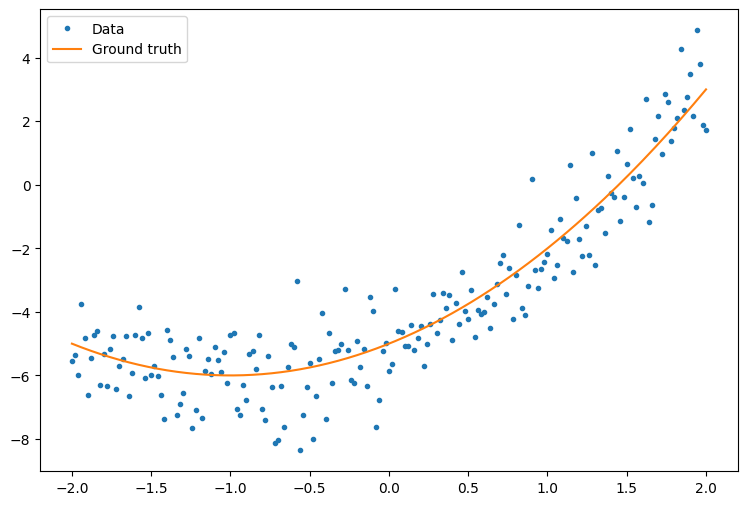Create a quadratic model with randomly initialized weights and a bias:

class Model(tf.Module):

def __init__(self):
# Randomly generate weight and bias terms
rand_init = tf.random.uniform(shape=, minval=0., maxval=5., seed=22)
# Initialize model parameters
self.w_q = tf.Variable(rand_init)
self.w_l = tf.Variable(rand_init)
self.b = tf.Variable(rand_init)

@tf.function
def __call__(self, x):
# Quadratic Model : quadratic_weight * x^2 + linear_weight * x + bias
return self.w_q * (x**2) + self.w_l * x + self.b


First, observe your model's performance before training:

quad_model = Model()

def plot_preds(x, y, f, model, title):
plt.figure()
plt.plot(x, y, '.', label='Data')
plt.plot(x, f(x), label='Ground truth')
plt.plot(x, model(x), label='Predictions')
plt.title(title)
plt.legend()

plot_preds(x, y, f, quad_model, 'Before training')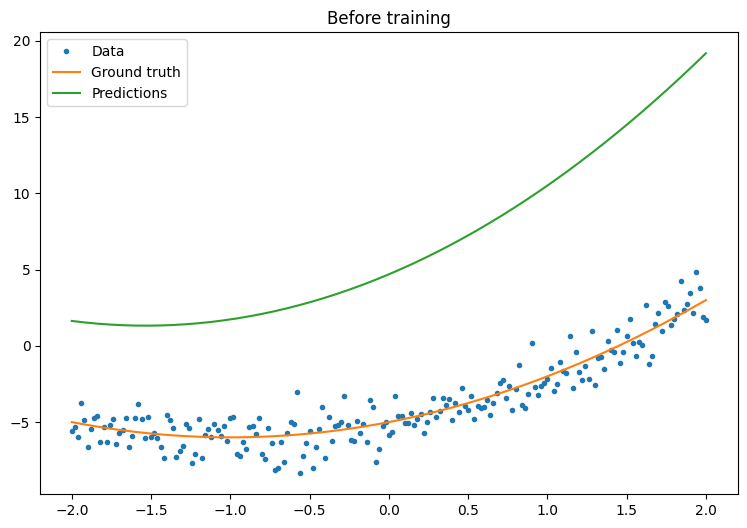Now, define a loss for your model:

Given that this model is intended to predict continuous values, the mean squared error (MSE) is a good choice for the loss function. Given a vector of predictions, $$\hat{y}$$, and a vector of true targets, $$y$$, the MSE is defined as the mean of the squared differences between the predicted values and the ground truth.

$$MSE = \frac{1}{m}\sum_{i=1}^{m}(\hat{y}_i -y_i)^2$$

def mse_loss(y_pred, y):
return tf.reduce_mean(tf.square(y_pred - y))


Write a basic training loop for the model. The loop will make use of the MSE loss function and its gradients with respect to the input in order to iteratively update the model's parameters. Using mini-batches for training provides both memory efficiency and faster convergence. The tf.data.Dataset API has useful functions for batching and shuffling.

batch_size = 32
dataset = tf.data.Dataset.from_tensor_slices((x, y))
dataset = dataset.shuffle(buffer_size=x.shape).batch(batch_size)

# Set training parameters
epochs = 100
learning_rate = 0.01
losses = []

# Format training loop
for epoch in range(epochs):
for x_batch, y_batch in dataset:
# Update parameters with respect to the gradient calculations
v.assign_sub(learning_rate*g)
# Keep track of model loss per epoch
losses.append(loss)
if epoch % 10 == 0:
print(f'Mean squared error for step {epoch}: {loss.numpy():0.3f}')

# Plot model results
print("\n")
plt.plot(range(epochs), losses)
plt.xlabel("Epoch")
plt.ylabel("Mean Squared Error (MSE)")
plt.title('MSE loss vs training iterations');

Mean squared error for step 0: 55.997
Mean squared error for step 10: 9.566
Mean squared error for step 20: 3.970
Mean squared error for step 30: 2.031
Mean squared error for step 40: 1.366
Mean squared error for step 50: 1.127
Mean squared error for step 60: 1.039
Mean squared error for step 70: 1.010
Mean squared error for step 80: 0.999
Mean squared error for step 90: 0.996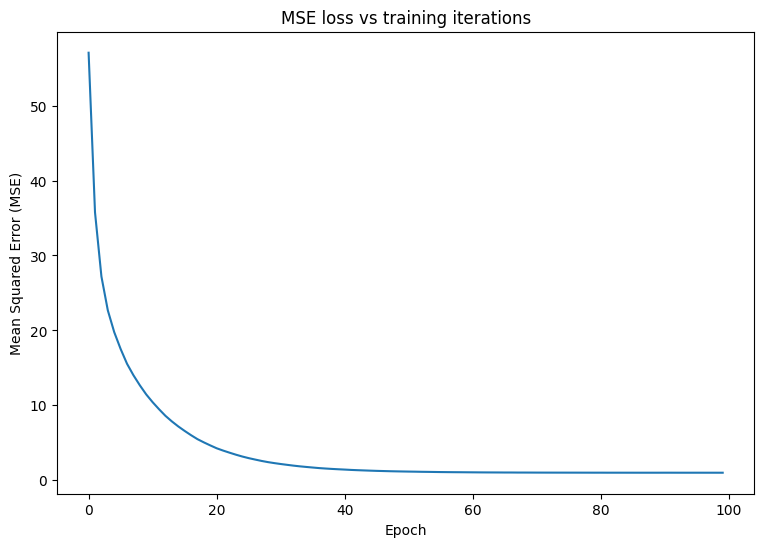Now, observe your model's performance after training:

plot_preds(x, y, f, quad_model, 'After training')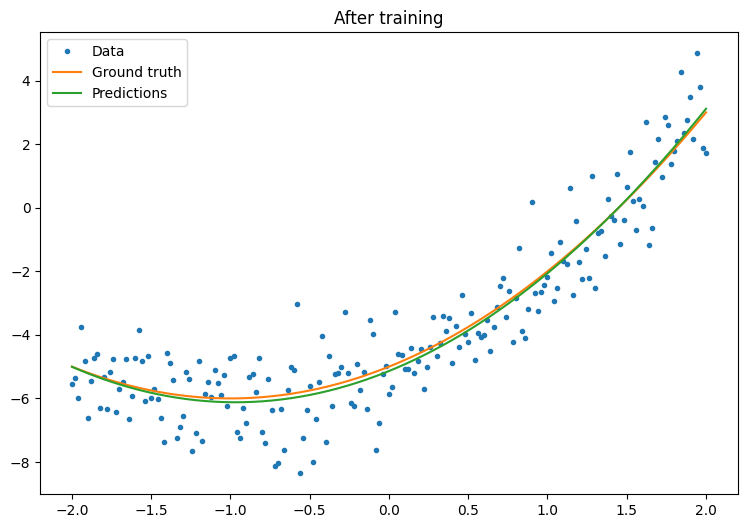That's working, but remember that implementations of common training utilities are available in the tf.keras module. So, consider using those before writing your own. To start with, the Model.compile and Model.fit methods implement a training loop for you:

Begin by creating a Sequential Model in Keras using tf.keras.Sequential. One of the simplest Keras layers is the dense layer, which can be instantiated with tf.keras.layers.Dense. The dense layer is able to learn multidimensional linear relationships of the form $$\mathrm{Y} = \mathrm{W}\mathrm{X} + \vec{b}$$. In order to learn a nonlinear equation of the form, $$w_1x^2 + w_2x + b$$, the dense layer's input should be a data matrix with $$x^2$$ and $$x$$ as features. The lambda layer, tf.keras.layers.Lambda, can be used to perform this stacking transformation.

new_model = tf.keras.Sequential([
tf.keras.layers.Lambda(lambda x: tf.stack([x, x**2], axis=1)),
tf.keras.layers.Dense(units=1, kernel_initializer=tf.random.normal)])

new_model.compile(
loss=tf.keras.losses.MSE,
optimizer=tf.keras.optimizers.SGD(learning_rate=0.01))

history = new_model.fit(x, y,
epochs=100,
batch_size=32,
verbose=0)

new_model.save('./my_new_model')

INFO:tensorflow:Unsupported signature for serialization: ((TensorSpec(shape=(2, 1), dtype=tf.float32, name='gradient'), <tensorflow.python.framework.func_graph.UnknownArgument object at 0x7fabb7fa0d30>, 140375502898560), {}).
INFO:tensorflow:Unsupported signature for serialization: ((TensorSpec(shape=(2, 1), dtype=tf.float32, name='gradient'), <tensorflow.python.framework.func_graph.UnknownArgument object at 0x7fabb7fa0d30>, 140375502898560), {}).
INFO:tensorflow:Unsupported signature for serialization: ((TensorSpec(shape=(1,), dtype=tf.float32, name='gradient'), <tensorflow.python.framework.func_graph.UnknownArgument object at 0x7fabb7f4f550>, 140375502941760), {}).
INFO:tensorflow:Unsupported signature for serialization: ((TensorSpec(shape=(1,), dtype=tf.float32, name='gradient'), <tensorflow.python.framework.func_graph.UnknownArgument object at 0x7fabb7f4f550>, 140375502941760), {}).
INFO:tensorflow:Unsupported signature for serialization: ((TensorSpec(shape=(2, 1), dtype=tf.float32, name='gradient'), <tensorflow.python.framework.func_graph.UnknownArgument object at 0x7fabb7fa0d30>, 140375502898560), {}).
INFO:tensorflow:Unsupported signature for serialization: ((TensorSpec(shape=(2, 1), dtype=tf.float32, name='gradient'), <tensorflow.python.framework.func_graph.UnknownArgument object at 0x7fabb7fa0d30>, 140375502898560), {}).
INFO:tensorflow:Unsupported signature for serialization: ((TensorSpec(shape=(1,), dtype=tf.float32, name='gradient'), <tensorflow.python.framework.func_graph.UnknownArgument object at 0x7fabb7f4f550>, 140375502941760), {}).
INFO:tensorflow:Unsupported signature for serialization: ((TensorSpec(shape=(1,), dtype=tf.float32, name='gradient'), <tensorflow.python.framework.func_graph.UnknownArgument object at 0x7fabb7f4f550>, 140375502941760), {}).
INFO:tensorflow:Assets written to: ./my_new_model/assets
INFO:tensorflow:Assets written to: ./my_new_model/assets


Observe your Keras model's performance after training:

plt.plot(history.history['loss'])
plt.xlabel('Epoch')
plt.ylim([0, max(plt.ylim())])
plt.ylabel('Loss [Mean Squared Error]')
plt.title('Keras training progress');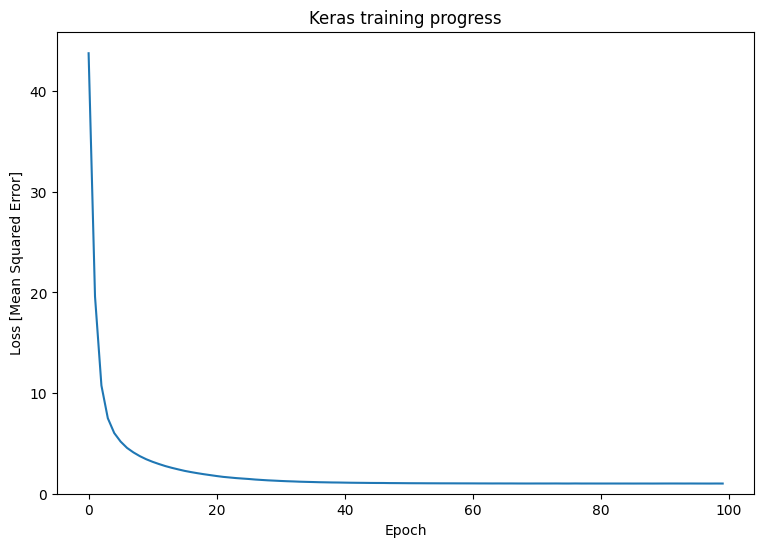plot_preds(x, y, f, new_model, 'After Training: Keras')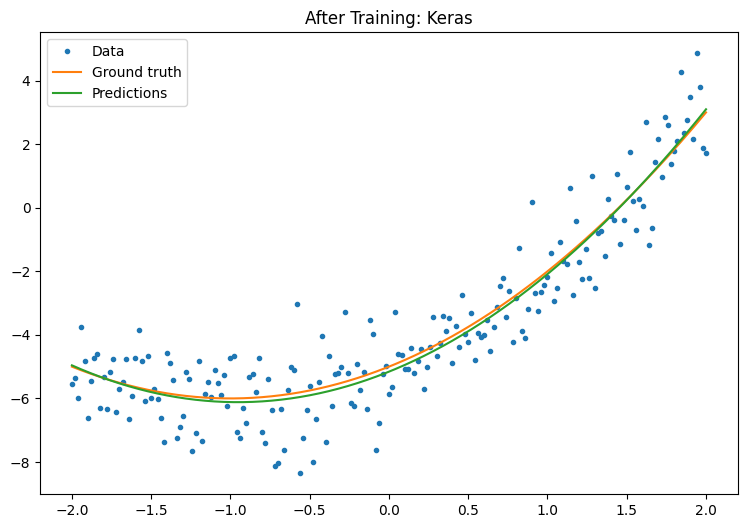Refer to Basic training loops and the Keras guide for more details.

[]
[]